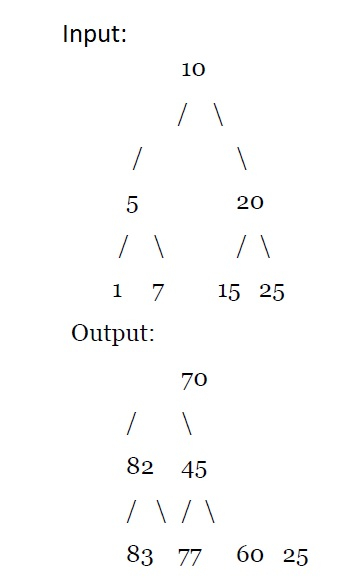# Add all greater values to every node in a given BST in C++ ?

A BST or binary search tree is a form of binary tree that has all left nodes smaller and all right nodes greater than the root value. For this problem, we will take a binary tree and add all the values greater than the current node to it. the problem “ add all greater values to every node in BST” is simplified as for a BST add all the node values that are greater than the current node value to that node value.

Add all greater values to each node in BST Problem Statement −

Given a Binary Search Tree (BST), we need to add to each node, the sum of all greater values node.## Explanation

This program will convert a BST into a Binary Tree with a value of nodes as the sum of all greater elements plus the original value of the node.

Add all greater values to each node in Binary Search Tree solution −

We use Reverse Inorder Traversal (recursion is called on right subtree first rather than left subtree) and maintain a variable to store the sum of nodes that have been traversed so far.

We then use this sum to modify the value of our present node, by first adding its value to the sum, and then replacing the value of the node with this sum.

## Example

#include <iostream >
using namespace std;
struct node{
int data;
node *left;
node *right;
};
node *newNode(int key){
node *temp=new node;
temp->left=NULL;
temp->right=NULL;
temp->data=key;
return temp;
}
void Inorder(node *root){
if(!root)
return;
Inorder(root->left);
cout<<root->data<<" ";
Inorder(root->right);
}
node *Insert(node *root,int key){
if(!root)
return newNode(key);
if(key<root->data)
root->left=Insert(root->left,key);
else
root->right=Insert(root->right,key);
return root;
}
if(!root)
return;
sum+=root->data;
root->data=sum;
}
int sum=0;
}
int main() {
/* Let us create following BST
10
/ \
5 20
/ \ / \
1 7 15 25 */
node *root = NULL;
root = Insert(root, 10);
Insert(root, 20);
Insert(root, 25);
Insert(root, 15);
Insert(root, 5);
Insert(root, 7);
Insert(root, 1);
Inorder(root);
cout<<endl;
}